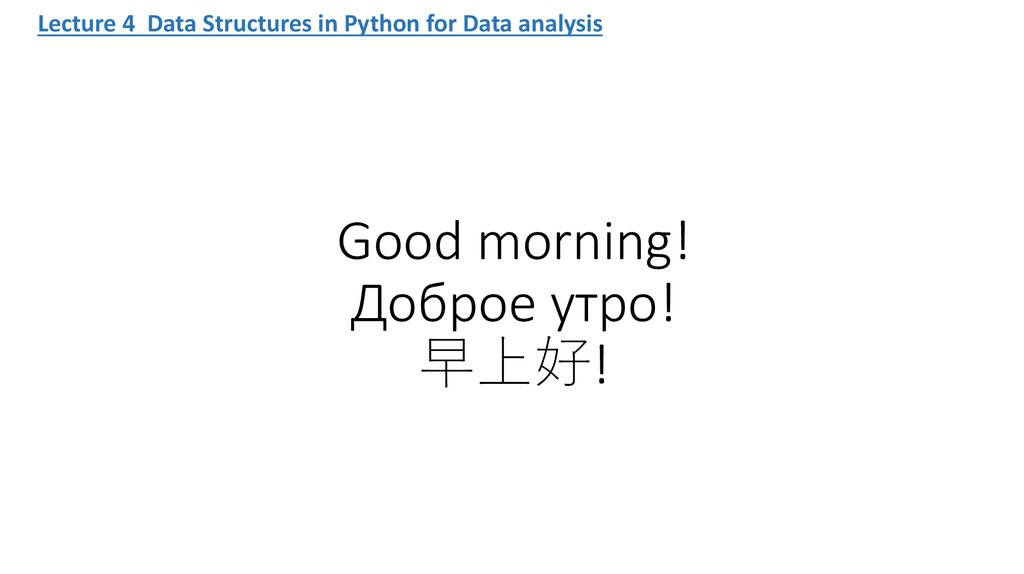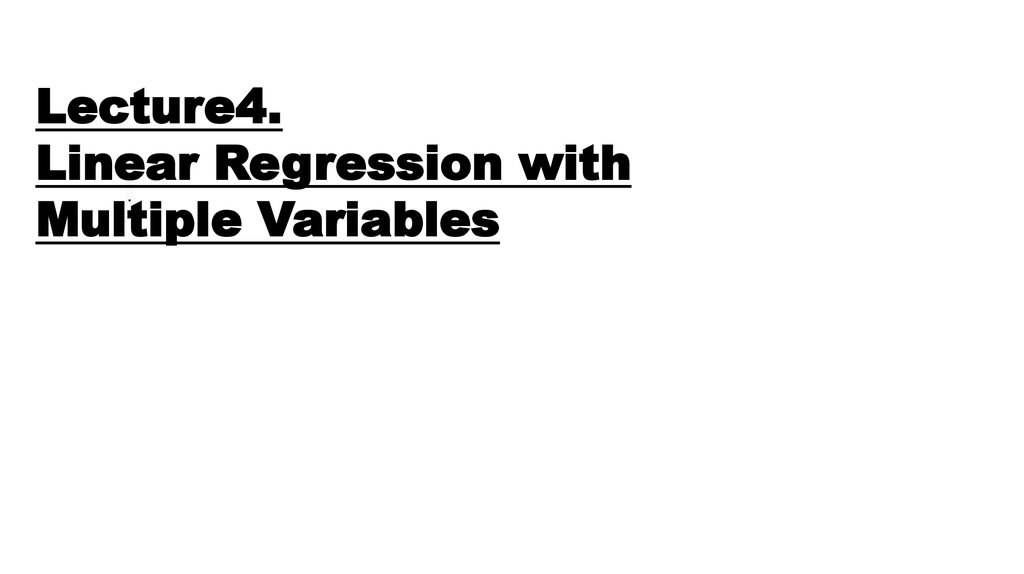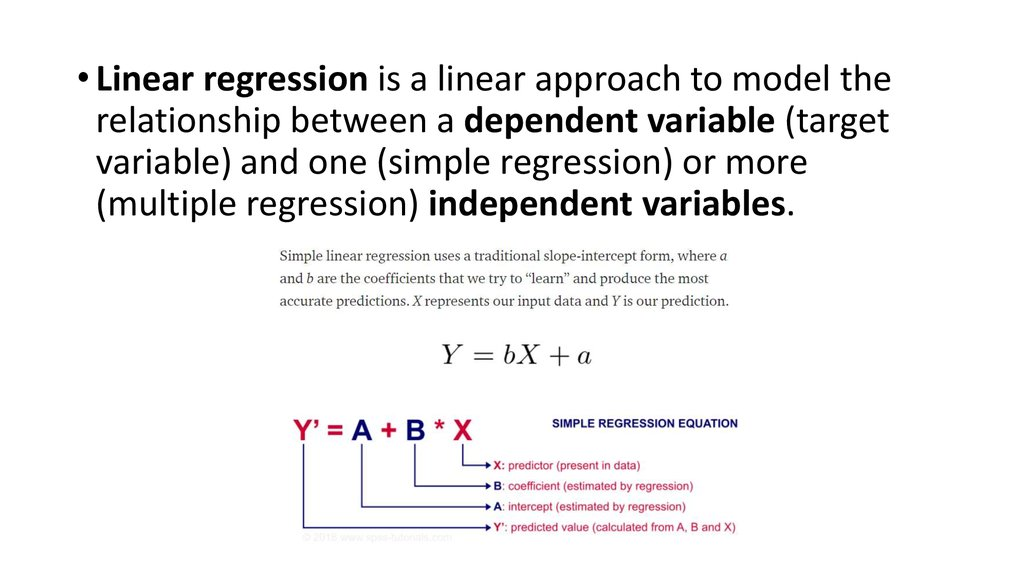# Linear regression with multiple variables

## 1. Good morning! Доброе утро! 早上好!

Lecture 4 Data Structures in Python for Data analysis
Good morning!
Доброе утро!

## 2. .

Lecture4.
Linear
Regression
with
.
Multiple Variables

## 3.

• Linear regression is a linear approach to model the
relationship between a dependent variable (target
variable) and one (simple regression) or more
(multiple regression) independent variables.

## 4.

the model shows the dependence of salary on seniority. if we train the
model, she will predict salary

## 16.

• Example
http://localhost:8890/notebooks/Regression%20for%20heightweight.ipynb

## 17. This is a link to the lecture. You now need to view it, preferably using headphones. There are subtitles in Chinese here.

• https://www.coursera.org/lecture/machinelearning/model-representation-db3jS
• https://www.coursera.org/learn/machinelearning/home/week/1

## 21. watch the video lecture

• https://www.coursera.org/lecture/machinelearning/what-is-machine-learning-Ujm7v
• https://www.coursera.org/learn/machinelearning/lecture/6Nj1q/multiple-features

## 22.

• Numerical variables represent values that can be measured and
sorted in ascending and descending order such as the height of a
person.
• Categorical variables are values that can be sorted in groups or
categories such as the gender of a person.
• Multiple linear regression accepts not only numerical variables, but
also categorical ones. To include a categorical variable in a regression
model, the variable has to be encoded as a binary variable (dummy
variable).

## 23. Preprocessing Data If data set are strings

• We saw in our initial exploration that most of the columns in our data
set are strings, but the algorithms in scikit-learn understand only
numeric data. Luckily, the scikit-learn library provides us with many
methods for converting string data into numerical data. One such
method is the LabelEncoder() method. We will use this method to
convert the categorical labels in our data set like ‘won’ and ‘loss’ into
numerical labels. To visualize what we are trying to to achieve with
the LabelEncoder() method let’s consider the images below.

## 24.

• The image below represents a dataframe that has one column named ‘color’ and
three records ‘Red’, ‘Green’ and ‘Blue’.
• Since the machine learning algorithms in scikit-learn understand only numeric
inputs, we would like to convert the categorical labels like ‘Red, ‘Green’ and ‘Blue’
into numeric labels. When we are done converting the categorical labels in the
original dataframe, we would get something like this